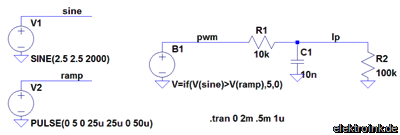## 12. December 2008 Generate Modulated PWM with LTSpice

LTSpice knows different signal sources. Unfurtunately there is no modulated PWM. This article shows how to generate a voltage controlled PWM.At first we need two normal voltage sources “voltage”. One voltage source generates the modulation voltage, the other generates the clock for the PWM.

For this example we want to generate a PWM with 20 kHz clock that is modulated by a sine wave of 2000 Hz.

As modulation source every other voltage form is usable too.The voltage source that generates the sine wave will be set acordingly to the requirements. The PWM shall be 5 V peak voltage and single ended (ground based). That’s why the sine gets an offset voltage of 2.5 V and an amplitude of 2.5 V. That causes the PWM to a middle value of 50%. The maximum modulation of the PWM depends only on the amplitude of the sine wave. At 2.5 V amplitude the modulation will be 100%.The second voltage source generates the clock of the PWM. To achieve an actual PWM this voltage source must generate a triangular voltage. For 20 kHz the period duration is 50 µs, one ramp has a duration of 25 µs. The amplitude of this source is also set to 5 V.

Up to now this is not a PWM yet. Now both voltages need to be connected.For this a common ground needs to be defined and each voltage needs a unique name. The voltage sources are connected to ground with their “-” terminal. The “+” terminal is connected to a short wire. These wires are open on the other end. Each wire is given a unique name. In our example they are named “sine” and “ramp”.

Now only the combination of both voltages to a PWM is missing.

For that combination we use the “arbitrary behavioural voltage source”. These voltage sources generate a voltage that is defined by formulas and functions.

For our use a simple IF-function will suffice: if the value of the sine wave is higher than the triangular voltage a voltage will be put out. If the value is lower 0 will be put out. For our example we choose 5 V for the peak value of the PWM.

This voltage source must be connectet to ground too. That the PWM does not stand for itself the output is connected to a simple RC low pass filter.

The last figure shows all voltages as the result.

• Red: the sine wave,
• Blue: the triangular voltage,
• Green: the PWM and
• Yellow: the voltage from the PWM after the low pass filter(No Ratings Yet)Loading...
© 2006 — 2019 Transistorgrab.de

### 15 Kommentare »

1. Hy,

thanks for this small tutorial
very usefull for me to simulate an DC/AC converter with a MOSFETs bridge

Sincerely
Thomas

Kommentar by Thomas —